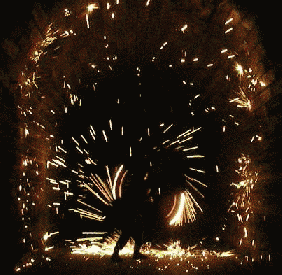# The King Needs Your Help! (Posted in Honour of Otto Bretscher)The King of Randomia has a problem. He is the proud father of three sons: Bingo, Toto and Lotto. Only one of the sons can succeed him as king but they are all suitable to rule the kingdom. At his wit's end he decides to use the following method to decide who his successor will be:

At a succession ceremony, the king will toss a coin repeatedly until two consecutive heads or two consecutive tails come up.

Bingo will become king if it is two successive heads $(...HH)$ and this occurs in an even number of tosses.

Toto will become king if it is two tails $(...TT)$ and this occurs in an even number of tosses.

Lotto will become king if it is either two heads $(...HH)$ or two tails $(...TT)$ and this occurs in an odd number of tosses.

The subjects are in an uproar because they think this method of selection is not fair.

Investigate the situation and decide whether or not the sons have an equal chance of becoming the next king. Will this spark a debate (reason for image)?

This problem was posed by Bertus Van Etten of the Institute for Mathematics and Science Teaching of the University of Stellenbosch.Note by A Former Brilliant Member
6 years, 2 months ago

This discussion board is a place to discuss our Daily Challenges and the math and science related to those challenges. Explanations are more than just a solution — they should explain the steps and thinking strategies that you used to obtain the solution. Comments should further the discussion of math and science.

When posting on Brilliant:

• Use the emojis to react to an explanation, whether you're congratulating a job well done , or just really confused .
• Ask specific questions about the challenge or the steps in somebody's explanation. Well-posed questions can add a lot to the discussion, but posting "I don't understand!" doesn't help anyone.
• Try to contribute something new to the discussion, whether it is an extension, generalization or other idea related to the challenge.

MarkdownAppears as
*italics* or _italics_ italics
**bold** or __bold__ bold
- bulleted- list
• bulleted
• list
1. numbered2. list
1. numbered
2. list
Note: you must add a full line of space before and after lists for them to show up correctly
paragraph 1paragraph 2

paragraph 1

paragraph 2

[example link](https://brilliant.org)example link
> This is a quote
This is a quote
    # I indented these lines
# 4 spaces, and now they show
# up as a code block.

print "hello world"
# I indented these lines
# 4 spaces, and now they show
# up as a code block.

print "hello world"
MathAppears as
Remember to wrap math in $$ ... $$ or $ ... $ to ensure proper formatting.
2 \times 3 $2 \times 3$
2^{34} $2^{34}$
a_{i-1} $a_{i-1}$
\frac{2}{3} $\frac{2}{3}$
\sqrt{2} $\sqrt{2}$
\sum_{i=1}^3 $\sum_{i=1}^3$
\sin \theta $\sin \theta$
\boxed{123} $\boxed{123}$

Sort by:

@Brian Charlesworth I would love to hear what you think!

- 6 years, 2 months ago

Interesting question. At first glance I'm getting equal probabilities for all three, but given that this is supposed to spark a debate makes me think I'm missing something. Looking at the possible sequences, Bingo and Toto have identical situations from a probability standpoint, each with one scenario of winning after any $2n$ throws, $n \ge 1.$ Thus the probability for each of them is

$\displaystyle\sum_{n=1}^{\infty} \left(\dfrac{1}{2}\right)^{2n} = \dfrac{\frac{1}{4}}{1 - \dfrac{1}{4}} = \dfrac{1}{3}.$

So the probability that Lotto will succeed is $1 - 2*\dfrac{1}{3} = \dfrac{1}{3}$ as well. Just as a check, we note that Lotto has two ways of winning after any odd number of throws $\ge 3,$ which will have a probability of

$\displaystyle 2*\sum_{n=1}^{\infty} \left(\dfrac{1}{2}\right)^{2n+1} = \sum_{n=1}^{\infty} \left(\dfrac{1}{2}\right)^{2n} = \dfrac{1}{3},$

thus confirming the previous observation.

- 6 years, 2 months ago

Too many o's can be a cause for mistakes- I think you got lotto and toto mixed up.

- 6 years, 2 months ago

Haha, Yeah, it can. :P Thanks for catching that mix-up; in my mind I had them in alphabetical order, but looking back now that wasn't the case.

- 6 years, 2 months ago

On this spreadsheet, we can see that, earlier on, the chances of each brother being chosen are nearly the same and we can also see that the probability of alternate heads and tails and no result being decided gets closer and closer to zero and the probabilities for each brother being chosen get closer to one third.

To find the total probability for Bingo being chosen we need to sum the following infinite geometric series (and the same series applies to the probability of Toto being chosen).

Probability of Bingo$=\frac{1}{4}+\frac{1}{4}^2+\frac{1}{4}^3+\frac{1}{4}^4+...=\frac{\frac{1}{4}}{1-\frac{1}{4}}=\frac{1}{3}$.

As the probability of the process going on for ever with no decision is $lim_{n→∞}\frac{1}{4}^n=0$ it follows that each brother has a probability of one third of being chosen.

Therefore, you are correct! (P.S.Try this as well. It seems nobody can solve it).

- 6 years, 2 months ago

What's their family motto? :D

- 6 years, 2 months ago

$\color{#D61F06}{\text{God does not play dice.}}$

$\color{#20A900}{\text{He flips coins!}}$

- 6 years, 2 months ago

He plays a mean game of poker, too. :)

- 6 years, 2 months ago